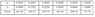# Physichal chemistry ideal solutions

• demander

#### demander

how can we without any calculation see if a solutions is ideal or non ideal?
for exemple if you are given a sort of values for pressure and for each the vapour and liquid composition of a mixture
how can we say if there is a ideal or non ideal solution?
i'm getting crazy with this cause normally when i saw something about ideal solutions i always see some calculations, so how can i, only looking to values as i said above, say if a solution is non ideal or ideal?
can be seen by dispertion of values? conjugation of values?
how?

maybe for some this question is too easy but after a week of electrochemistry i really have my brain stuck :S and i can't answer this.

i didn't put this on homework section cause my main problem is the theory behind this, but if you see this is in the wrong section be free to move.

Last edited:

One can assess a solutions ideality by calculating the excess molar volume due to adding two liquids for instance ethanol and water ; one way of finding out the excess molar volume is through a method involving refractive index measurements and some data plotting. I can describe this method subsequently if needed.

for example giving the table in attachment with the values where x is the liquid compound and y the vapour compound of the same solution how can i say that the solution is non ideal without calculations? can a diagram be usefull in that situation? and if you can please explain that method you talk before

#### Attachments

•table.JPG
16.1 KB · Views: 377
Alright you want to utilize Raoult's law for the diagram - it really depends on what "P" is - is it the total P? Assuming that the parameter for x and y is mole fraction it seems that the solution itself is non-deal since both the mole fraction of the vapor P and the vapor P itself is not linear with respect to its relationship with the mole fraction in the solution - regardless you need to inform me on what P actually is as well as the other parameters in the solution.

x and y are exactly what you said mole fraction of each component( liquid (x) vapour (y)
P is the vapour pressure of the diferent compositions of liquid and gas
of a mixture of ethanol + chlorofom at 45ºC (318,15K), don't know if this last line matters for it or not but i put it to complete the information

hope i have said what i want, sorry for my english a bit confusing :S

Last edited:
So the P is the vapor pressure of both ethanol and chloroform? Irregardless use Raoult's equation to construct a diagram of the mole fraction of the analyte on the x-axis and the vapor P of that analyte on the y-axis - you should observe a straight line. The line should be linear; any kind of curvature indicates non ideality.

ok thanks very much for the help, was really really usefullby the way connected with this same question what is in fact the(or an) azeotropic point? i see in many places but was all a bit confusing seem that is the pressure at what the boiling doesn't change anything... but how can this be aplied to activity coeficients
?